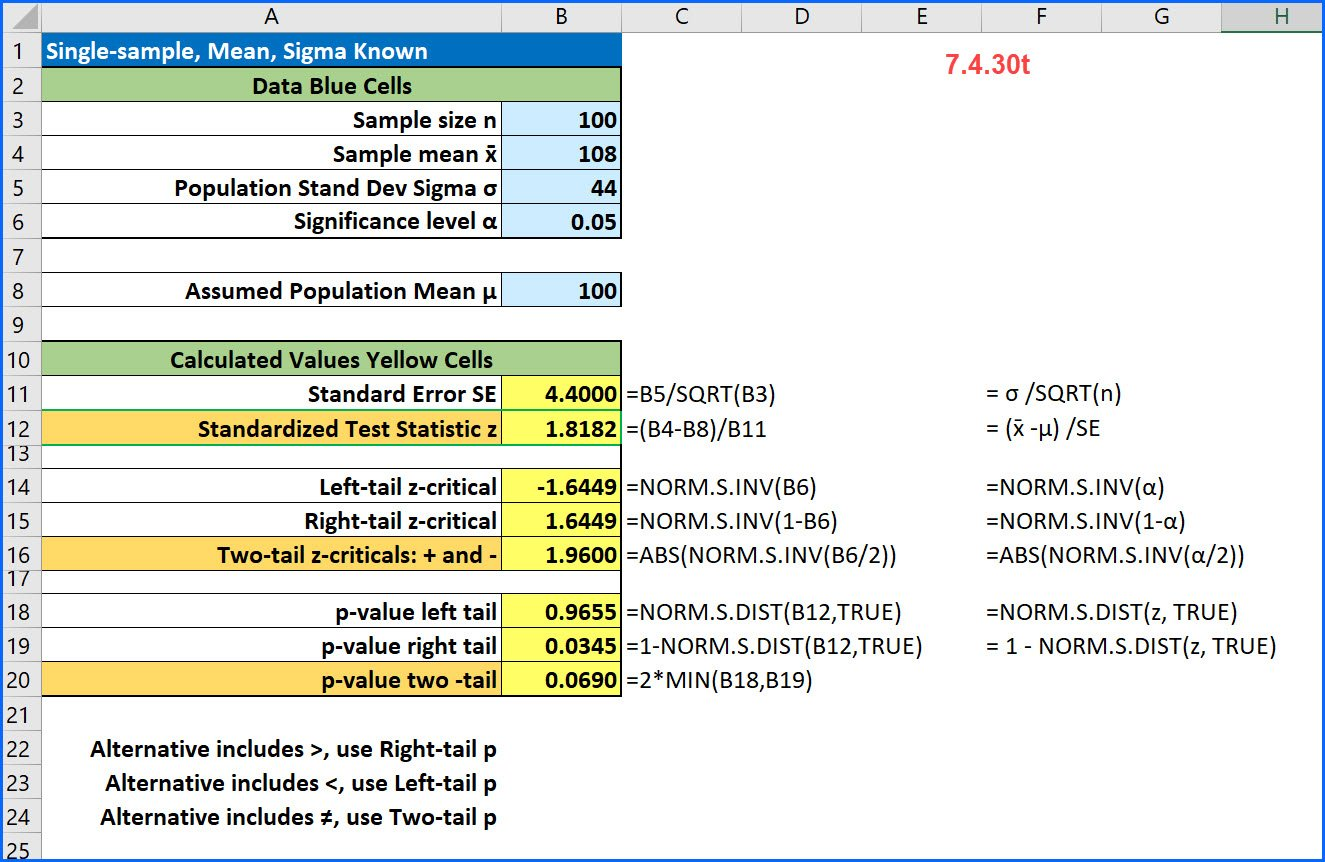# Dawn Wright

Ph.D. in Instructional Design and Development. Teaching statistics for Excelsior College and consulting. Zoomie class of '67.This is the Excel solution using slightly different values:

## What is the Correlation Coefficient?

This is an excerpt from a longer, 12-minute video by Unbreaking America. I used it as an example of regression on a current event. I wonder how many Americans are data savvy enough to understand what Lawrence is showing them. I asked my students to identify the predictor variable and the response variable and to …## Single-sample z-test for the mean [7.4.30t]

Here is a common problem from intro stats: [7.4.30t] A random sample of 100 observations from a population with a standard deviation of 44 yielded a sample mean of 108. Test the null hypothesis that μ = 100 against the alternative that μ > 100 at an alpha of 0.05. Here because the alternative contains …

## Picking Alpha: The cost of Type I and Type II Errors-2

Earlier this year, I read of the analysis of the recent fatality caused by an Uber autonomous car in Arizona. (Lee, 2018) It occurred to me (and others) that this terrible situation is possibly related to Type I and Type II errors. (Efrati, 2018) A Type I is a false positive where a true null …

## The Big Picture of Statistics

The Big Picture of Statistics The process of statistics starts when we identify what group we want to study or learn something about. We call this group the population. Note that the word “population” here (and in the entire course) is not just used to refer to people; it is used in the broader statistical …## Why is the Standard Error Equal to Sigma Divided by the Square Root of n?

Photo by Ken Treloar on UnsplashEvery time I teach the Central Limit Theorem, I get questions from students on why we divide the population standard deviation, sigma, by the square root of the sample size to calculate the standard deviation of the sampling distribution which we call the standard error. Recall that the equation for …

Scroll to Top